Dr. J's Maths.com
Where the techniques of Maths
are explained in simple terms.

Test Yourself 1.

Answer the following questions - you will need to use the discriminant:

CALCULATE THE VALUE

INTERPRET directly

Find values

 No real roots. 1.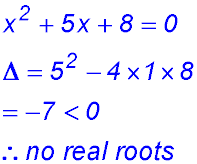2.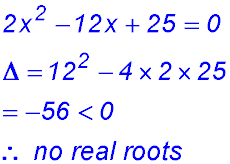3.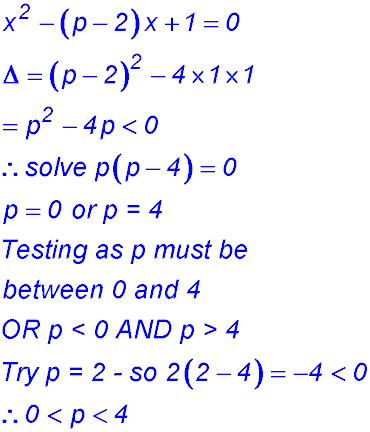4.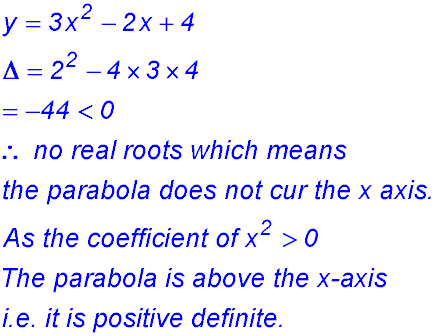Real roots 5.6. (i)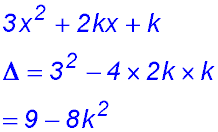(ii)7.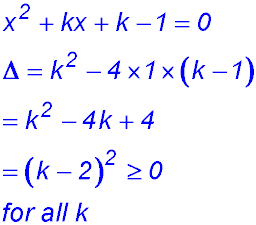8. One real root 9.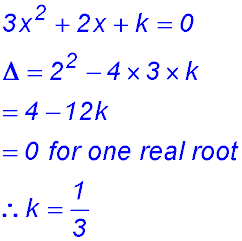10.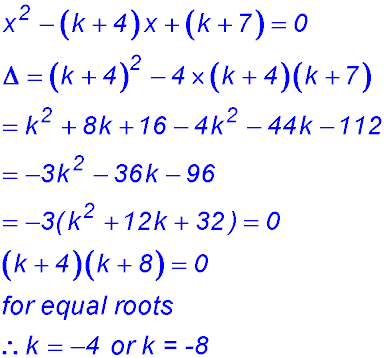11.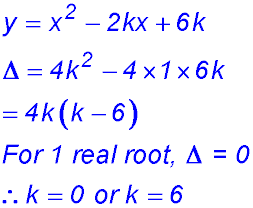12. Real and different roots. 13.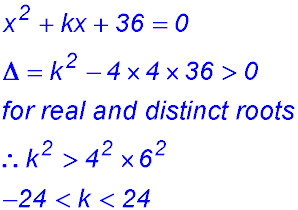14. 15.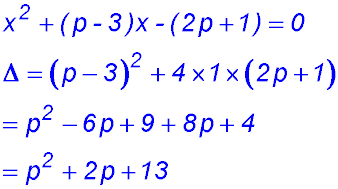(ii) To have real, distinct roots for real valued p, the discriminant must be > 0.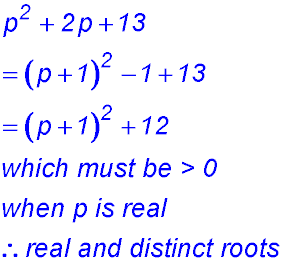16. Mixed questions. 17.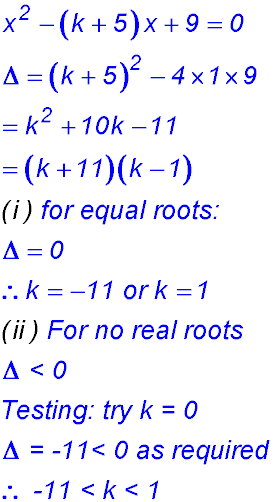Positive definite. 19.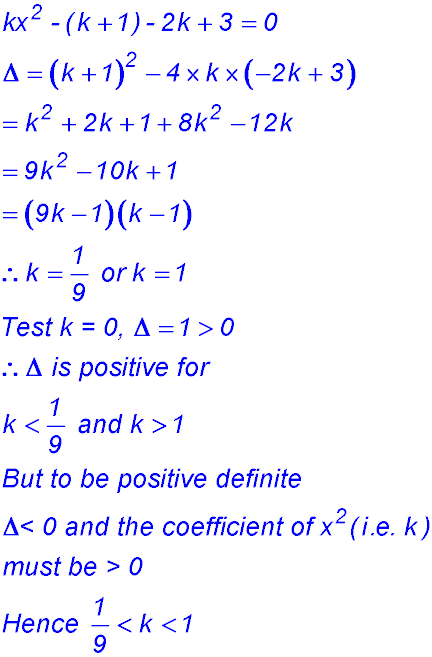20.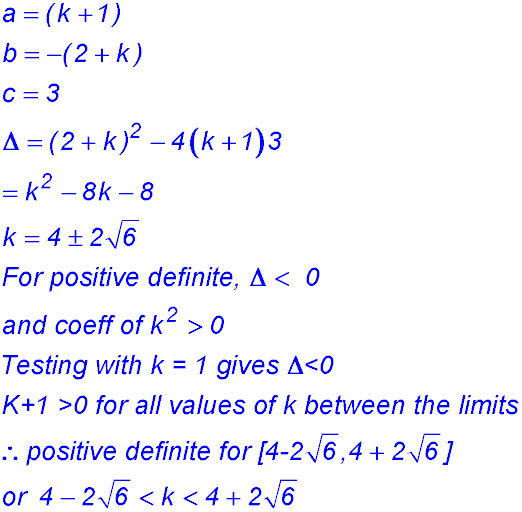21. Negative definite. 23. Advanced contexts. 27. (i)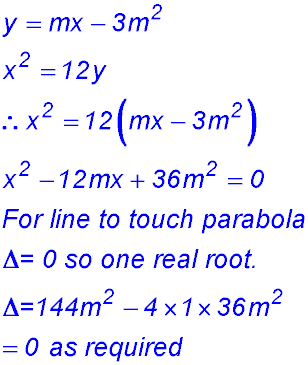(ii) Find the values of m for which this line passes through the point (5,2). (iii) Hence determine the equations of the two tangents to the given parabola from the point (5,2). 28. The circle x2 + (y – c)2 = r2, where c > 0 and r > 0, lies inside the parabola y = x2. The circle touches the parabola at exactly two points located symmetrically on opposite sides of the y-axis, as shown in the diagram.   (i) Show that 4c = 1 + 4r2 (ii) Deduce that c > ½. 29.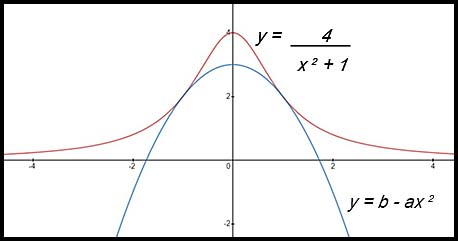(i)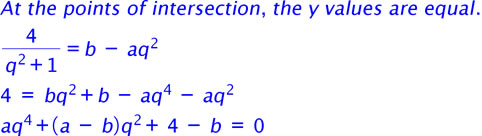(ii)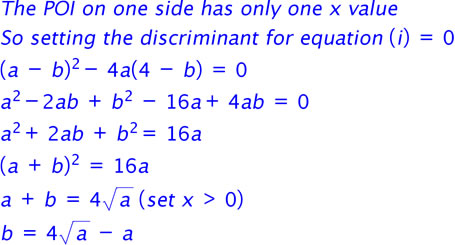(iii)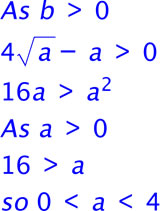26. (i) Write down the discriminant of 2x2 + (k –2)x + 8, where k is a constant. (ii) Hence, or otherwise, find the values of k for which the parabola y =2x2 + kx + 9 does not intersect the line y =2x +1. Increasing functions. For what values of k is the curve y = x3 - 3x2 + kx + 3 always an increasing function? Decreasing functions.